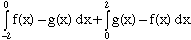Subject: Graphing regions Name: Esther Who is asking: Parent Level: Secondary Question: This is the second of two questions my son had problems with. Find the area of the region enclosed by the graphs of y = x3-6x and y = -2x between their points of intersection. Hi Esther, Let f(x) = x3-6x and g(x) = -2x. First you need to find where the curves intersect. Solving f(x) = g(x) gives x3 - 6x = -2x x(x2 - 4) = 0 x = -2, 0 or 2 Thus the curves cross at three points, where x = -1, 0 or 2. Now between x = -2 and x = 0 and between x = 0 and x = 2 you need to determine "which curve is on top and which is on the bottom", that is which function f or g is larger. Choose a point between x = -2 and x = 0, say x = -1. f(-1) = (-1)3 -6(-1) = 5 and g(-1) = -2(-1) = 2 Thus between -2 and 0 f is larger than g. Choose a point between x = 0 and x = 2, say x = 1. f(1) = (1)3 -6(1) = -5 and g(-1) = -2(1) = -2 Thus between 0 and 2 g is larger than f. Hence the area between the two curves isCheers, Harley Go to Math Central